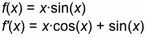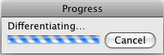# Define Derivative FunctionThis Number menu command creates a new function that is the derivative of the selected function with respect to that function’s independent variable.

Derivative functions update automatically when the function they differentiate is edited.

In most ways derivative functions behave just like other functions you create. You can calculate with derivative functions, evaluate derivative functions for specific arguments, and plot derivative functions. You can even create the derivative of a derivative. The only thing you can’t do with derivative functions is to edit them directly; you must edit the original function instead.Differentiation of complicated functions can be time-consuming. In these cases, a dialog box like the one at right appears. Click Cancel if you want to interrupt computation of an exact derivative. If you cancel the computation, Sketchpad will provide you with an approximate derivative, in the formThis approximate derivative can still be plotted, and can even be differentiated itself. Although there is some loss in accuracy, in most cases the approximation resembles the exact derivative.

The approximate derivative is always used to find the derivative of a function defined by a drawing or picture.

See Sketchpad’s Internal Mathematics for a more detailed discussion of derivatives.